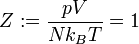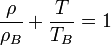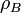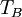# Difference between revisions of "Zeno line"This article is a 'stub' page, it has no, or next to no, content. It is here at the moment to help form part of the structure of SklogWiki. If you add sufficient material to this article then please remove the {{Stub-general}} template from this page.

The Zeno line is the name given to a line along which the compressibility factor is unity$Z:= \frac{pV}{Nk_BT}=1$

## Batchinsky law

The Batchinsky law  states that:$\frac{\rho}{\rho_B} + \frac{T}{T_B} = 1$

where$\rho_B$ is the value of the density obtained by the extrapolating the coexistence curve into the low temperature region beyond the triple point, and$T_B$ is the Boyle temperature.